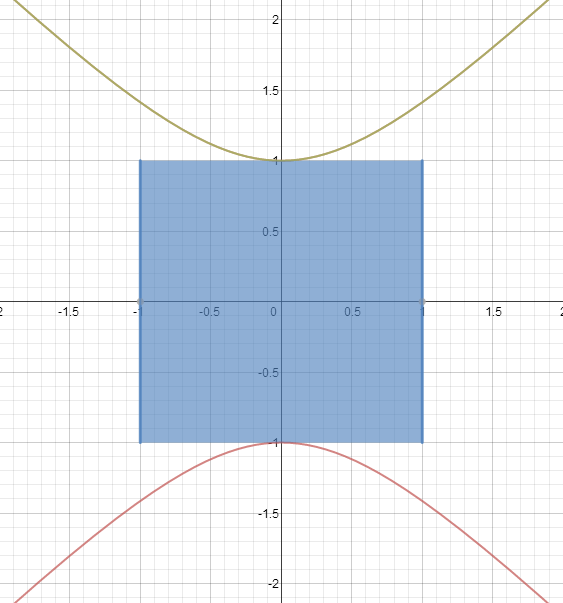# Smallest Square Tangent to a Hyperbola

Calculus Level 5The smallest possible side length of a square who has 2 opposite sides that are tangential to the hyperbola $y^2=x^2+1$ can be represented as $1+S$. What is $\lfloor 1000S\rfloor$?

Details and Assumptions
The square given in the picture does not possess the shortest possible side length.
Having two opposite corners touch the hyperbola does not count as having the square tangent to it. The two opposite sides must be tangent.

×